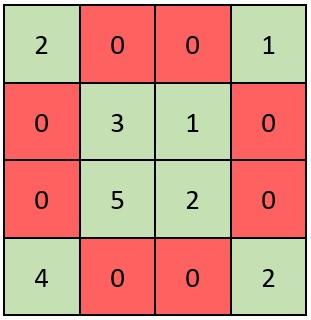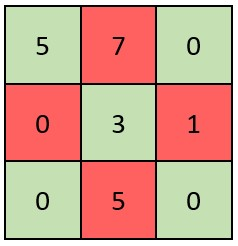2319. Check if Matrix Is X-Matrix
Easy
398
17

A square matrix is said to be an X-Matrix if both of the following conditions hold:

1. All the elements in the diagonals of the matrix are non-zero.
2. All other elements are 0.

Given a 2D integer array `grid` of size `n x n` representing a square matrix, return `true` if `grid` is an X-Matrix. Otherwise, return `false`.

Example 1:```Input: grid = [[2,0,0,1],[0,3,1,0],[0,5,2,0],[4,0,0,2]]
Output: true
Explanation: Refer to the diagram above.
An X-Matrix should have the green elements (diagonals) be non-zero and the red elements be 0.
Thus, grid is an X-Matrix.
```

Example 2:```Input: grid = [[5,7,0],[0,3,1],[0,5,0]]
Output: false
Explanation: Refer to the diagram above.
An X-Matrix should have the green elements (diagonals) be non-zero and the red elements be 0.
Thus, grid is not an X-Matrix.
```

Constraints:

• `n == grid.length == grid[i].length`
• `3 <= n <= 100`
• `0 <= grid[i][j] <= 105`
Accepted
40.2K
Submissions
60.6K
Acceptance Rate
66.3%

Seen this question in a real interview before?
1/4
Yes
No

Discussion (0)

Related Topics